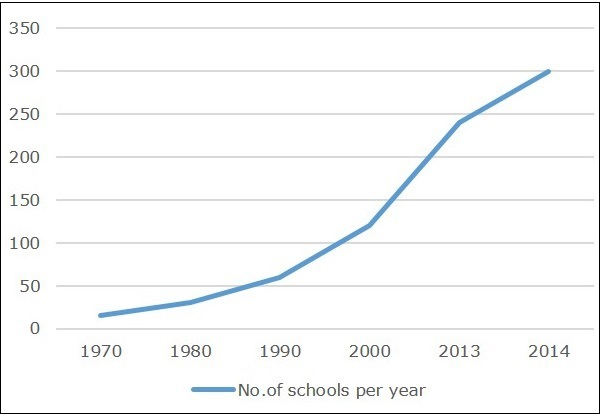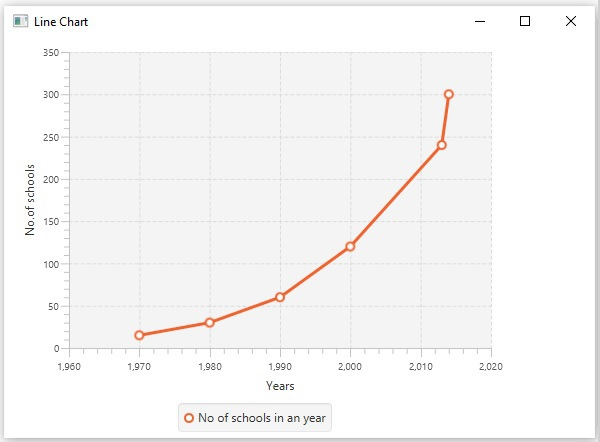# JavaFX - Line Chart

A line chart or line graph displays information as a series of data points (markers) connected by straight line segments. A Line Chart shows how the data changes at equal time frequency.

Following is a Line chart depicting the number of schools in different years.In JavaFX, a line chart is represented by a class named LineChart. This class belongs to the package javafx.scene.chart. By instantiating this class, you can create a LineChart node in JavaFX.

## Steps to Generate Line Chart

To generate a line chart in JavaFX, you should follow the steps given below.

### Step 1: Creating a Class

Create a Java class and inherit the Application class of the package javafx.application. You can then implement the start() method of this class as follows.

```public class ClassName extends Application {
@Override
public void start(Stage primaryStage) throws Exception {
}
}
```

### Step 2: Defining the axis

Define the X and Y axis of the line chart and set labels to them.

In our example, the X axis represent the years starting from 1960 to 2020 having major tick mark at every ten years.

```//Defining X axis
NumberAxis xAxis = new NumberAxis(1960, 2020, 10);
xAxis.setLabel("Years");

//Defining y axis
NumberAxis yAxis = new NumberAxis(0, 350, 50);
yAxis.setLabel("No.of schools");
```

### Step 3: Creating the Line Chart

Create a line chart by instantiating the class named LineChart of the package javafx.scene.chart. To the constructor of this class, pass the objects representing the X and Y axis created in the previous step.

```LineChart linechart = new LineChart(xAxis, yAxis);
```

### Step 4: Preparing the Data

Instantiate the XYChart.Series class. Then add the data (a series of, x and y coordinates) to the Observable list of this class as follows −

```XYChart.Series series = new XYChart.Series();
series.setName("No of schools in an year");

```

### Step 5: Add Data to the Line Chart

Add the data series prepared in the previous step to the line chart as follows −

```//Setting the data to Line chart
```

### Step 6: Creating a Group Object

In the start() method, create a group object by instantiating the class named Group. This belongs to the package javafx.scene.

Pass the LineChart (node) object, created in the previous step as a parameter to the constructor of the Group class. This should be done in order to add it to the group as follows −

```Group root = new Group(linechart);
```

### Step 7: Creating a Scene Object

Create a Scene by instantiating the class named Scene, which belongs to the package javafx.scene. To this class, pass the Group object (root), created in the previous step.

In addition to the root object, you can also pass two double parameters representing height and width of the screen along with the object of the Group class as follows.

```Scene scene = new Scene(group ,600, 300);
```

### Step 8: Setting the Title of the Stage

You can set the title to the stage using the setTitle() method of the Stage class. The primaryStage is a Stage object, which is passed to the start method of the scene class as a parameter.

Using the primaryStage object, set the title of the scene as Sample Application as follows.

```primaryStage.setTitle("Sample Application");
```

### Step 9: Adding Scene to the Stage

You can add a Scene object to the stage using the method setScene() of the class named Stage. Add the Scene object prepared in the previous steps using this method as follows.

```primaryStage.setScene(scene);
```

### Step 10: Displaying the Contents of the Stage

Display the contents of the scene using the method named show() of the Stage class as follows.

```primaryStage.show();
```

### Step 11: Launching the Application

Launch the JavaFX application by calling the static method launch() of the Application class from the main method as follows.

```public static void main(String args[]){
launch(args);
}
```

### Example

The following table depicts the number of schools that were in an area from the year 1970 to 2014.

Year Number of Schools
1970 15
1980 30
1990 60
2000 120
2013 240
2014 300

Following is a Java program which generates a line chart, depicting the above data, using JavaFX.

Save this code in a file with the name LineChartExample.java.

```import javafx.application.Application;
import javafx.scene.Group;
import javafx.scene.Scene;
import javafx.stage.Stage;
import javafx.scene.chart.LineChart;
import javafx.scene.chart.NumberAxis;
import javafx.scene.chart.XYChart;

public class LineChartExample extends Application {
@Override
public void start(Stage stage) {
//Defining the x axis
NumberAxis xAxis = new NumberAxis(1960, 2020, 10);
xAxis.setLabel("Years");

//Defining the y axis
NumberAxis yAxis = new NumberAxis   (0, 350, 50);
yAxis.setLabel("No.of schools");

//Creating the line chart
LineChart linechart = new LineChart(xAxis, yAxis);

//Prepare XYChart.Series objects by setting data
XYChart.Series series = new XYChart.Series();
series.setName("No of schools in an year");

//Setting the data to Line chart

//Creating a Group object
Group root = new Group(linechart);

//Creating a scene object
Scene scene = new Scene(root, 600, 400);

//Setting title to the Stage
stage.setTitle("Line Chart");

stage.setScene(scene);

//Displaying the contents of the stage
stage.show();
}
public static void main(String args[]){
launch(args);
}
}
```

Compile and execute the saved java file from the command prompt using the following commands.

```javac LineChartExample.java
java LineChartExample
```

On executing, the above program generates a JavaFX window displaying a line chart as shown below.javafx_charts.htm